# SQL SELECT with DISTINCT on multiple columns

## DISTINCT on multiple columns

In SQL multiple fields may also be added with DISTINCT clause. DISTINCT will eliminate those rows where all the selected fields are identical.

Example: Sample SELECT statement

Here is a simple query on some selected columns in orders table where agent_code='A002'

SQL Code:

``````SELECT agent_code, ord_amount, cust_code, ord_num
FROM orders
WHERE agent_code='A002';
``````

Relational Algebra Expression: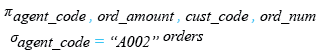Relational Algebra Tree: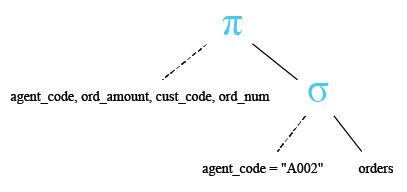Sample table: orders

Output:

```AGENT_CODE ORD_AMOUNT CUST_CODE     ORD_NUM
---------- ---------- ---------- ----------
A002             2500 C00005         200106
A002              500 C00022         200123
A002              500 C00009         200120
A002              500 C00022         200126
A002             3500 C00009         200128
A002             1200 C00009         200133
A002             4000 C00022         200113
```

The above result shows the same agent_code, ord_amount and cust_code appears more than once in theorders table.

Example: SELECT with DISTINCT on two columns

To get the identical rows (based on two columns agent_code and ord_amount) once from the orders table, the following SQL statement can be used :

SQL Code:

``````SELECT DISTINCT agent_code,ord_amount
FROM orders
WHERE agent_code='A002';
``````

Relational Algebra Expression: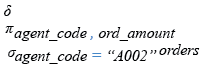Relational Algebra Tree: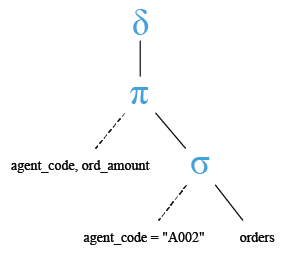Output:

```AGENT_CODE ORD_AMOUNT
---------- ----------
A002             3500
A002             1200
A002             4000
A002              500
A002             2500
```

Pictorial presentation: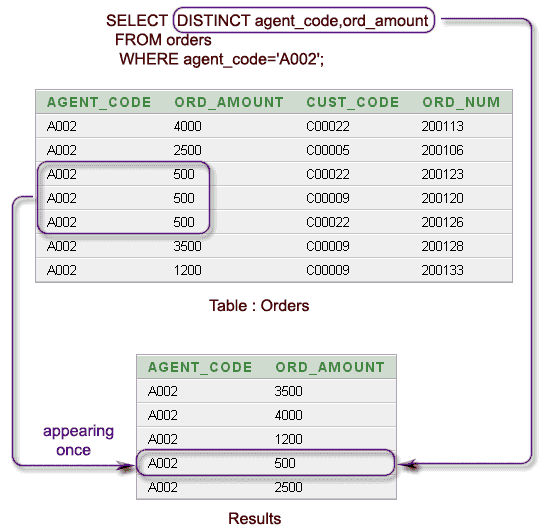Example: SELECT with DISTINCT on three columns

To get the identical rows (based on three columnsagent_code, ord_amount, and cust_code) once from the orders table, the following SQL statement can be used:

SQL Code:

``````SELECT DISTINCT agent_code, ord_amount,cust_code
FROM orders
WHERE agent_code='A002';
``````

Relational Algebra Expression: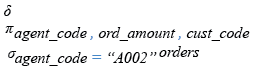Relational Algebra Tree: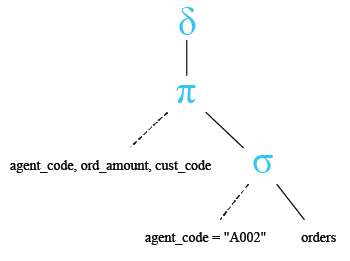Output:

```AGENT_CODE ORD_AMOUNT CUST_CODE
---------- ---------- ----------
A002              500 C00022
A002             3500 C00009
A002             2500 C00005
A002              500 C00009
A002             4000 C00022
A002             1200 C00009
```

Pictorial presentation:

Example : SELECT with DISTINCT on all columns of the first query

To get the identical rows (on four columns agent_code, ord_amount, cust_code, and ord_num) once from the orders table , the following SQL statement can be used :

SQL Code:

``````SELECT DISTINCT agent_code,ord_amount,cust_code,ord_num
FROM orders
WHERE agent_code='A002';
``````

Relational Algebra Expression: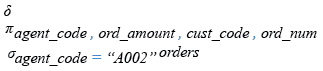Relational Algebra Tree: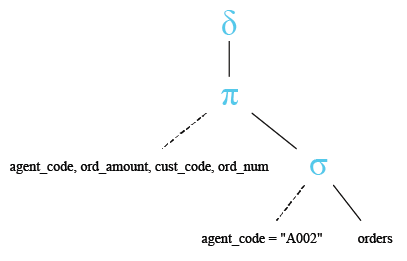Output:

```AGENT_CODE ORD_AMOUNT CUST_CODE     ORD_NUM
---------- ---------- ---------- ----------
A002              500 C00022         200126
A002             2500 C00005         200106
A002              500 C00009         200120
A002             1200 C00009         200133
A002             4000 C00022         200113
A002             3500 C00009         200128
A002              500 C00022         200123
```

In the above output, all rows whose agent_code is 'A002' have returned because there is no identical rows on agent_code, ord_amount, cust_code and ord_num. See the following presentation :

Pictorial presentation:

SELECT with DISTINCT on multiple columns and ORDER BY clause

You can use an order by clause in the select statement with distinct on multiple columns. Here is an example:

SQL Code:

``````SELECT DISTINCT agent_code,ord_amount
FROM orders
WHERE agent_code='A002'
ORDER BY ord_amount;
``````

Relational Algebra Expression: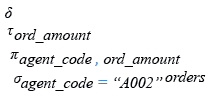Relational Algebra Tree: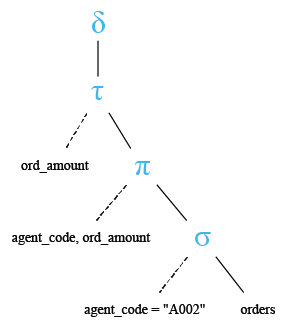Output:

```AGENT_CODE ORD_AMOUNT
---------- ----------
A002              500
A002             1200
A002             2500
A002             3500
A002             4000
```

Pictorial presentation: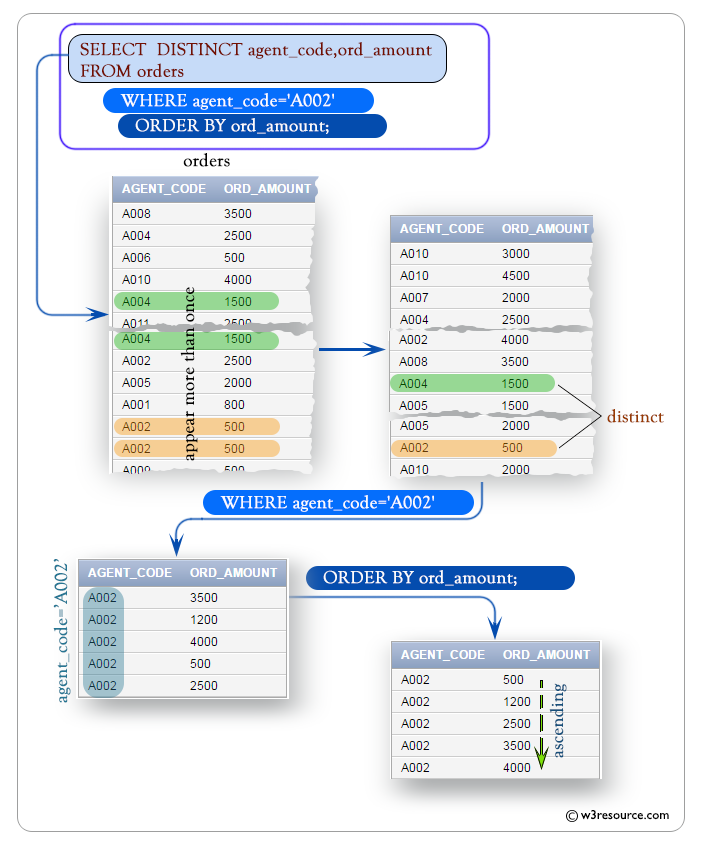COUNT() function and SELECT with DISTINCT on multiple columns

You can use the count() function in a select statement with distinct on multiple columns to count the distinct rows. Here is an example:

``````SELECT COUNT(*)
FROM (
SELECT  DISTINCT agent_code, ord_amount,cust_code
FROM orders
WHERE agent_code='A002');
``````

Output:

```  COUNT(*)
----------
6
```

Pictorial presentation:Practice SQL Exercises

﻿

## SQL: Tips of the Day

How to check if a table exists in a given schema?

```SELECT EXISTS (
SELECT FROM information_schema.tables
WHERE  table_schema = 'schema_name'
AND    table_name   = 'table_name'
);
```

Ref: https://bit.ly/3MjkoYD